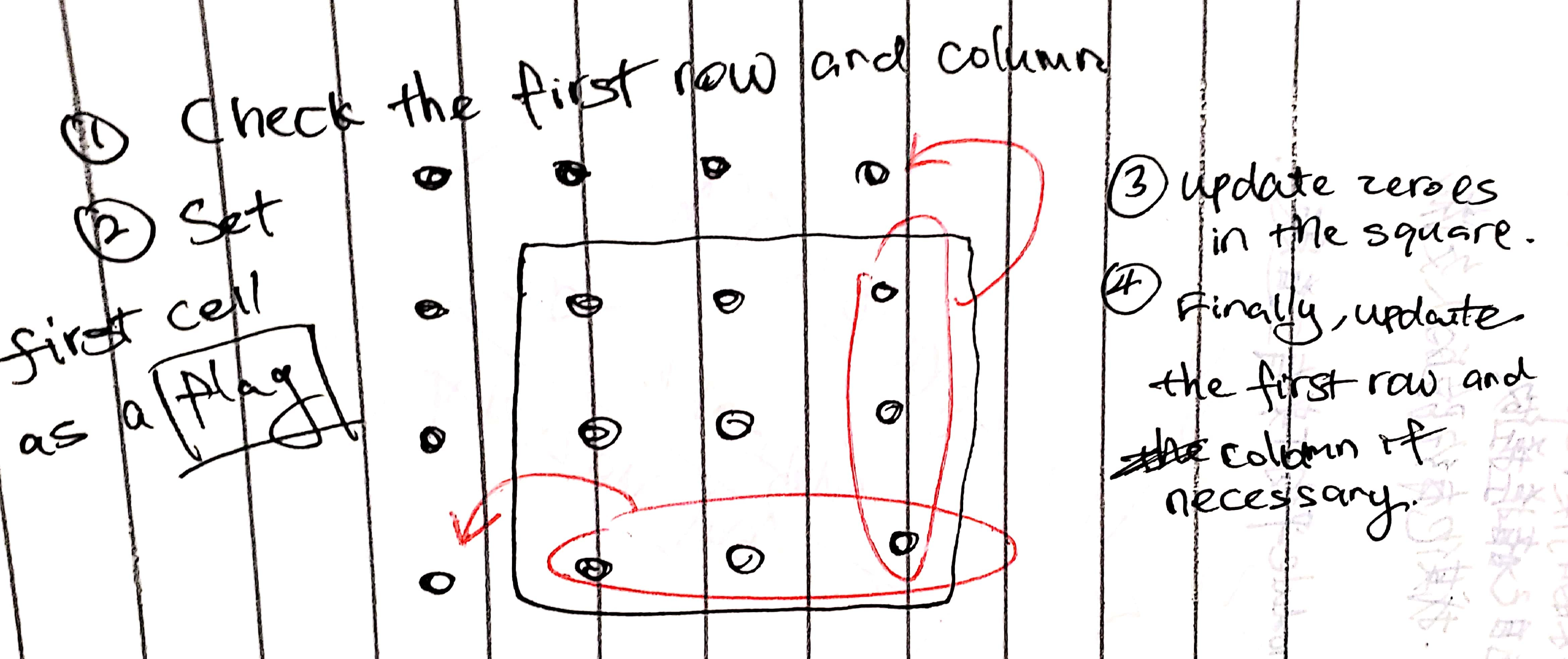# 73. Set Matrix Zeroes

Reference: LeetCode
Difficulty: Medium

## Problem

Given a m x n matrix, if an element is 0s, set its entire row and column to 0. Do it in-place.

Example:

• A straight forward solution using O(mn) space is probably a bad idea.
• A simple improvement uses O(m + n) space, but still not the best solution.
• Could you devise a O(1) space solution?

## Analysis

### Extra Space

Use two sets rows and cols to record which row and column should be set to zeroes.

Time: $O(MN)$
Space: $O(M + N)$

### Brute-Force + O(1) Space

We need two passes. The first pass is to set relevant non-zeroes to MODIFIED. The second pass is to set MODIFIED to 0.

Note: This value should not be in the matrix already; otherwise, this method won’t work.

Time: $O(MN \times (M + N))$
Space: $O(1)$

### Bette + O(1) SpaceTime: $O(MN)$
Space: $O(1)$

CommentJunhao Wang
a software engineering cat